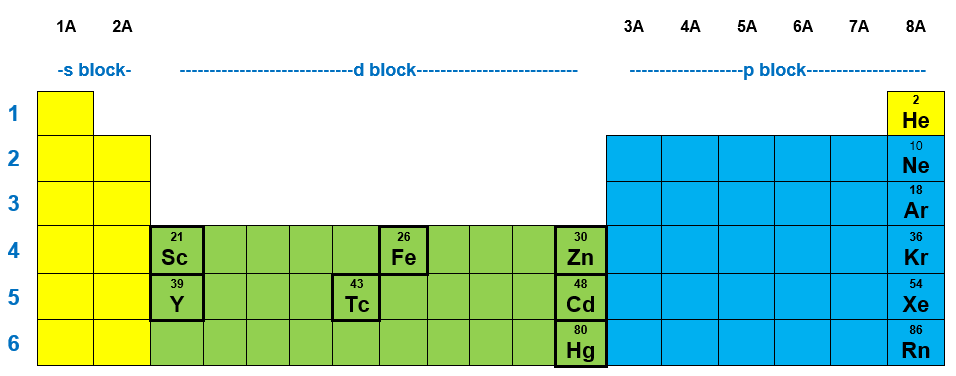# Problem: Which of these ions have one d electron in the outmost d subshell?a. Hg2+ b. Zn2+c. Y2+d. Fe2+ e. Cd2+ f. Sc2+ g. Tc2+

###### FREE Expert Solution

We’re being asked to determine the ion that would have one d electron in its outermost shell

Let us find these elements in the periodic table:The given elements belong to the d-block. However, we are given ions. We shall do the following:

Step 1: Identify the number of electrons in the ion.

Step 2: Write the electron configuration of the ion.

Step 3: Show the electrons in the outermost d-orbital.

90% (187 ratings)###### Problem Details

Which of these ions have one d electron in the outmost d subshell?

a. Hg2+

b. Zn2+

c. Y2+

d. Fe2+

e. Cd2+

f. Sc2+

g. Tc2+